# 18650 battery and 21700 battery comparison

The traditional 18650 battery has a mature supporting industrial chain and low cost. It is an ideal lithium-ion battery and has been widely used in the field of consumer electronics. However, the 18650 battery has a small capacity. When used in the field of power batteries, it often requires tens of thousands of single cells to be combined into a battery pack. This has led to a significant increase in the management difficulty of the battery pack. Therefore, under the leadership of Tesla, it has set off 21700 replaces the storm of 18650 batteries.

Compared with the 18650 battery, the 21700 battery has a higher capacity, reduces the number of single cells in the battery pack, reduces the complexity of the battery pack, and attracts a lot of attention. Recently, Thomas Waldmann (first author and corresponding author) of the Baden-Württemberg Solar Energy and Hydrogen Energy Research Center, Germany, and others conducted a comprehensive comparison of the two batteries in terms of electrical and thermal performance. The study showed that 21700 The battery produces more heat during high-current charging and discharging, so the polarization of the battery is correspondingly smaller. At the same time, the energy density can be increased by about 6% because the proportion of inactive materials such as the casing is reduced.

In order to be able to compare the two batteries more accurately, the author prepared 18650 and 21700 batteries with the same formula of electrodes, separators and electrolyte. The formula design of the two batteries is shown in the table below, so the main components of the two batteries are The difference comes from the size. In order to obtain the temperature information inside the battery in the experiment, the author implanted a K-type thermocouple in the middle of the battery.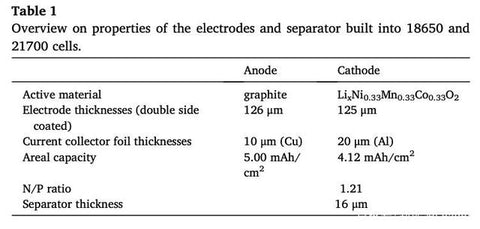The 18650 battery prepared by the author has a capacity of 1.625Ah, a weight of about 41g, and an internal resistance of 25mΩ, while the 21700 battery has a capacity of 2.457Ah, a weight of 59.71g, and an internal resistance of 13.2mΩ. The following figures a and b are the charge and discharge voltage curves of the two structures of the battery at different rates. It can be seen that the voltage curves of the two structures are very close, but at a higher rate, the discharge polarization of the 21700 battery is relatively Smaller, this is mainly because the internal resistance of the 21700 battery is smaller, and the temperature inside the battery is higher during the discharge process.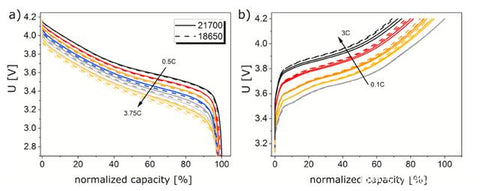From the relationship between the internal temperature of the battery and the battery discharge rate shown in the figure c below, it can be seen that as the battery charge and discharge rate increases, the internal temperature of the battery also increases significantly, but the 21700 battery with a larger diameter is at a high rate The lower temperature is significantly higher than the 18650 battery with a smaller diameter. At a rate of 3.5C, the internal temperature difference between the two batteries is 2.7°C. Calculations show that at this temperature difference, the diffusion coefficient of the graphite material inside the 21700 battery is 1.1 times that of the 18650 battery, which also explains why the 21700 battery is in The polarization is smaller when the discharge rate is large.

The following figure a shows the discharge capacity of the two batteries at different rates and the ratio of the discharge capacity of the two batteries. It can be seen that the two batteries have similar rate performance. From the discharge energy data shown in Figure b below, it can be seen that the 21700 battery has 50% more discharge energy than the 18650 battery. Taking into account the increase in the weight of the 21700 battery, the energy density of the battery is increased by about 6%.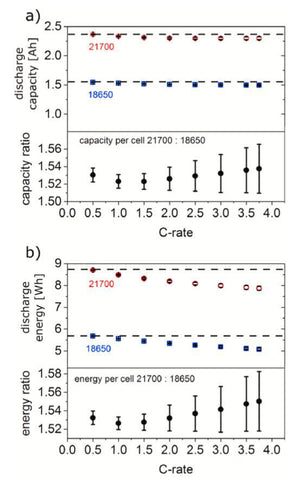In the figure below, the author uses CT technology to analyze the internal structure of the battery. According to the battery cross-sectional view obtained by CT, the author calculates the number of winding turns of the battery, including 20 turns for the 18650 battery and 26 turns for the 21700 battery.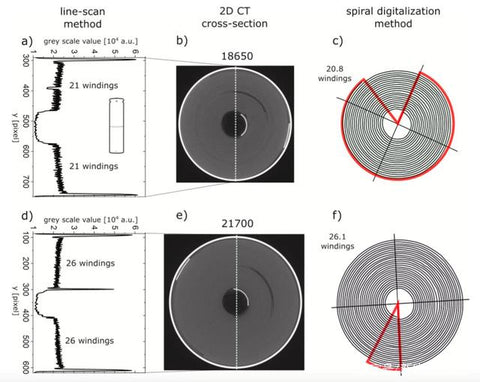According to the above CT data and calculation data, the 21700 battery is about 5.3 more turns of electrodes than the 18650 battery. Taking into account the change of the height of the 21700 battery, the overall capacity of the 21700 battery is about 51% more than that of the 18650 battery.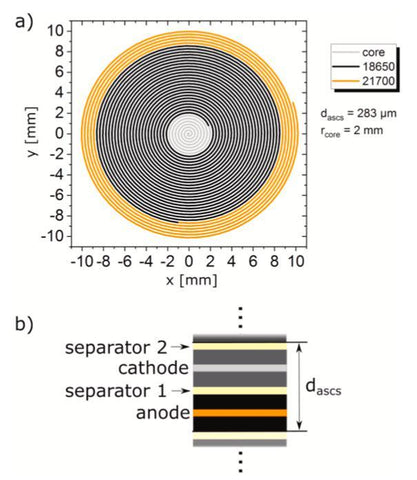For cylindrical batteries, there is a close relationship between the winding length and the electrode thickness. In the figure below, the author compares the difference of the thickness of the dascs (positive electrode thickness + negative electrode thickness + 2* diaphragm thickness) on the number of winding turns of the two batteries. As a whole, as the electrode thickness increases, the number of winding turns of the two batteries is decreasing, but basically the capacity increase of the 21700 battery due to the increase of the winding length and the increase of the battery height is about 50%.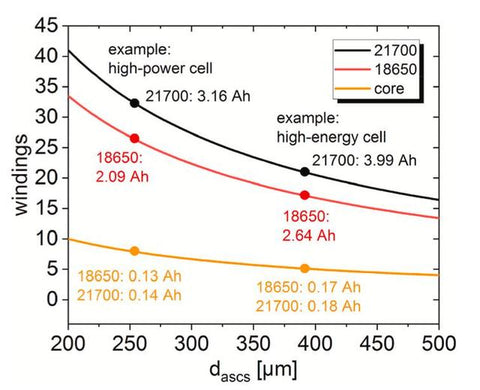Although the theoretical diameters of 21700 and 18650 batteries are 21mm and 18mm respectively, in fact, in order to be able to wind more electrodes and increase the capacity and energy density of the battery, the diameter of the energy-type battery is usually slightly larger than the theoretical value. For example, the author The measured outer diameter of a certain energy 21700 battery is actually about 21.15mm. Therefore, in the figure a below, the author analyzes the increased winding length of the battery when the outer diameter of the casing increases by 50-400um. From the figure, it can be seen that when the total thickness of the pole piece + diaphragm is 200um, when the diameter of the casing increases by 400um, the electrode is additionally increased. The length of the winding can reach 1 turn.

In the figure b below, the author compares the increased winding length of the battery when the outer diameter of the battery is 20700, 21700, 22700, 25700, and 30700 compared to the 18650 battery. It can be seen that when the total thickness of the electrode + diaphragm is 200um, The additional wound electrode of the 20700 battery increases by 5 turns, and the 21700 battery increases by 7.5 turns. Therefore, the capacity of the 18650 battery increases by 0.96Ah and 1.35Ah respectively. If you continue to increase the shell diameter to 22700, 25700 and 30700, the capacity will increase by 1.77Ah, respectively. 3.14Ah and 5.82Ah, with the increase of battery capacity, the proportion of inactive materials such as battery casing has decreased, so the specific energy of the battery has increased.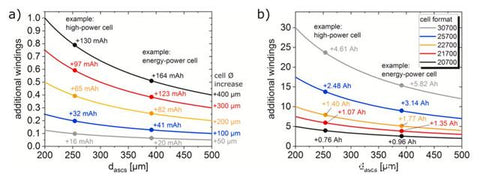The curvature of the pole piece is also of great significance to the winding structure of the cell. The following figure a shows the relationship between the electrode curvature and the number of winding turns under different electrode + diaphragm thicknesses. It can be seen that the closer to the middle of the cell The thinner the position and the total thickness of the electrode, the greater the curvature of the pole piece. This difference in curvature will also affect the electrode itself. The part of the electrode facing the shell is subjected to tension, while the part facing the battery core is subjected to pressure, which will have a certain effect on the bonding force of the electrode active material. Studies have shown that in the battery after cycling, the closer the negative electrode is to the center, the thicker the electrode, and the peeling of the active material toward the center of the electrode is more serious.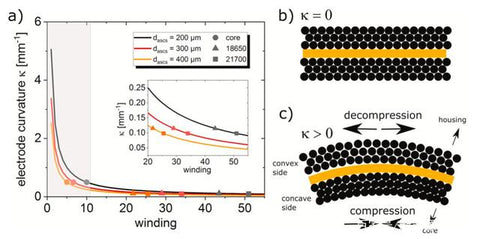The following figure shows the results of the cycling test of the 18650 and 21700 batteries at a rate of 1C. It can be seen that the capacity decay trends of the two batteries are basically the same, and the capacity decay rate is basically the same, which shows that under this test condition Below, the battery structure has less influence on the cycle performance of the battery, and the characteristics of the material itself have a greater influence on the cycle performance of the battery.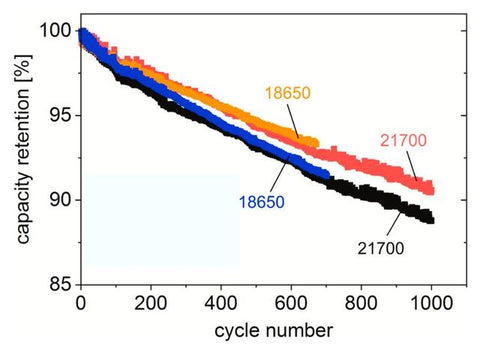Thomas Waldmann's research shows that the difference in structure will lead to differences in the electrical and thermal characteristics of the two batteries. For example, the 21700 battery has more obvious thermal effects during the discharge process, so the 21700 battery is less polarized in the rate test. The 21700 battery can involve more electrodes, so the weight of inactive parts such as the casing is significantly reduced, so the energy density of the battery is increased by 6% compared to the 18650 battery.